# Identition Zones (37-102)

Warning

This page is referring to child section which source is inherited by the prime spin with partitions listed below. Please return always to the Home Page to track the hierarchy.

Since the modulo 6 is occured all over the spin then we have defined that this 4 zones should stand as default configuration as you can see on the left sidebar.

## Extra dimensions

By this image you would see how the exponentiation zones should actually work on our solar system. But there is still not enough.

Note

Five consistent versions of superstring theory were developed before it was conjectured in the mid-1990s that they were all different limiting cases of a single theory in 11 dimensions known as M-theory (Wikipedia).Nowadays there are many scientists come in to the conclusion that there should be extra dimensions involved and typically it would take a very complicated form.

Note
1. Line/length
2. Plane/shapes
3. Depth (representing a stretching and shearing of the plane)
4. Time (stands as starting point to attemp the theory of everything)
5. Alternate world (we could measure similarities and differences of what might have been).
6. A plane of possible worlds that start with the same conditions (example: the Big Bang). Theoretically, if you were to master the sixth and seventh dimensions, you could travel through time.
7. Access to different worlds with different initial conditions. Here, everything would have happened differently, including the beginning conditions (one universe started with the Big Bang, another with the Oscillating Universe theory).
8. This dimension is similar to the seventh. There are multiple universes that all started differently and histories that branch out infinitely.
9. Here, we can compare all the could-have-been universes, each with a possibly different set of laws of physics.
10. Kinda like an extra room to accommodate ALL the theories. In additions, some physicists believe that at the instant of the Big Bang, the universe(s) was fully 10 dimensional.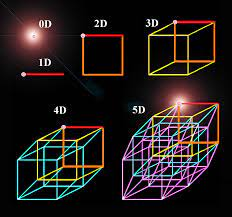The strong coupling dynamics of string theories in dimension d ⩾ 4 are studied. Some theories state that light is nothing but ripples of vibrations in the fifth dimension.

Note

It is argued, among other things, that eleven-dimensional supergravity arises as a low energy limit of the ten-dimensional Type IIA superstring, and that a recently conjectured duality between the heterotic string and Type IIA superstrings controls the strong coupling dynamics of the heterotic string in five, six, and seven dimensions and implies S-duality for both heterotic and Type II strings. (String Theory - Pdf)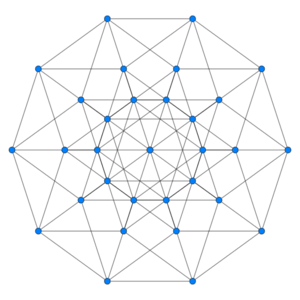In physics, string theory is a theoretical framework in which the point-like particles of particle physics are replaced by one-dimensional objects called strings.

Tip

The concept of eleven dimensions is a theoretical one in physics and cosmology, specifically in the realm of string theory and M-theory. These theories propose that our observable universe is made up of 11 dimensions, rather than the traditional three dimensions of length, width, and height, and the fourth dimension of time. The additional dimensions are thought to be compactified or curled up, meaning that they are not directly observable by us in our everyday experience. As for the cosmic philosophy, it is important to note that these theories are still considered speculative and have not been proven through experimental evidence. However, they do offer a new perspective on the nature of our universe and the fundamental forces that govern it. Some scientists and philosophers argue that these theories may provide new insights into the origins of the universe and the nature of reality itself. Ultimately, the concept of eleven dimensions is a fascinating area of study that continues to inspire new research and discoveries in the field of physics and cosmology. (ChatGPT)We would suggest that it is hidden in the imajinary part in the function of Euler Identity which brings the three (3) operations of addition, multiplication and exponentiation.

Note

We’ve seen how it [Euler’s identity] can easily be deduced from results of Johann Bernoulli and Roger Cotes, but that neither of them seem to have done so. Even Euler does not seem to have written it down explicitly – and certainly it doesn’t appear in any of his publications – though he must surely have realized that it follows immediately from his identity [i.e. Euler’s formula], e^ix = cos x + i sin x. Moreover, it seems to be unknown who first stated the result explicitly… (Wikipedia)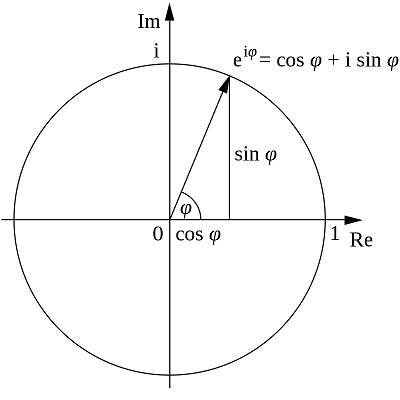Here we implement a method called the rank of a partition where the rank of a partition of a positive integer is a certain integer associated with the partition.

## Partition function

In mathematics, the rank of a partition is the number obtained by subtracting the number of parts in the partition from the largest part in the partition.

Note

The concept was introduced by Freeman Dysonin a paper published in the journal Eureka. It was presented in the context of a study of certain congruence properties of the partition function discovered by the Indian mathematical genius Srinivasa Ramanujan. (Wikipedia)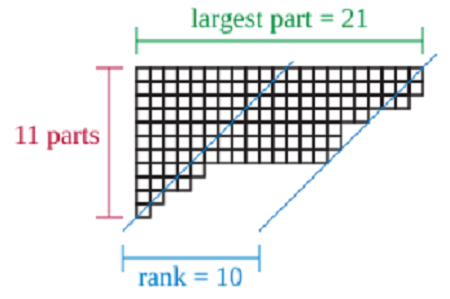In quantum field theory, partition functions are generating functionals for correlation functions, making them key objects of study in the path integral formalism.

Note

As usual, the images on the left are snapshots of the particles at different times. Those times correspond to the grey slices in the space-time diagram on the right. You can see the specific interaction points in the space-time diagram, where the blue particle is emitted and then absorbed by the red particles. Those points will be important later, as we compare this to the situation in string theory. (Slimy.com)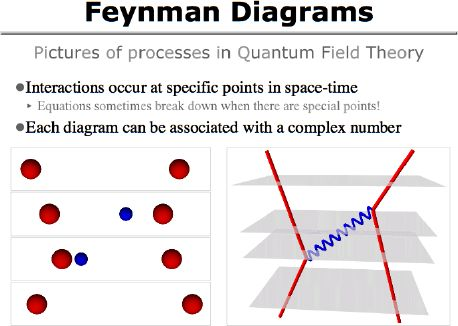One must therefore imagine scenarios in which these extra dimensions would not be observed in experiments so one of solution would be truncated approach.

Note

They are the imaginary time versions of statistical mechanics partition functions, giving rise to a close connection between these two areas of physics. Partition functions can rarely be solved for exactly, although free theories do admit such solutions. Instead, a perturbative approach is usually implemented, this being equivalent to summing over Feynman diagrams. (Wikiwand)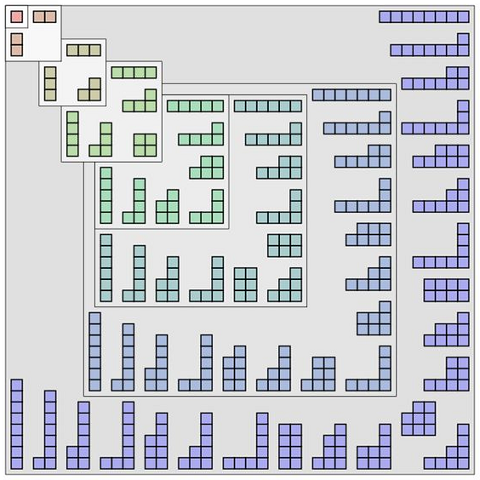Below is a model of E11 (shown by 11 dimensions). Its absolute dimensions represent all related key knowledges of modern physics. Moreover a model that represents Quark-Gluon Plasma, with fundamental forces in the early stage after Big Bang.

Note

It turns out that quantum string theory always destroys the symmetries of the classical string theory, except in one special case: when the number of dimensions is 10. That’s why string theory works only in 10 dimensions (Physicsforums).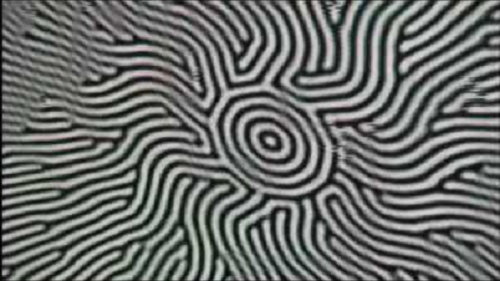In order to propagate through space and how they interact with each other we shall attemp it using string theory that bring the concept of eleven (11) dimensions.

## The powers of 10

Using this concept we are going to stimulate the 11 dimensions of string theory throug the rank of their partition using github organizations of 13 repositories each.

Tip

Each of the user profiles will have seven (7) user repositories consist of one (1) main of `github.io` and six (6) user pinned repositories. Meanwhile each of organizations will have one (1) profile of `.github` repository and thirteen (13) organization repositories consist of one (1) main of `github.io`, and twelve (12) pinned repositories under `member and public view` of six (6) repositories each.

7 + (11 x 1) + 7 + (11 x 13) = 24 x 7 = 168

1. "Scenarios"
• ["maps","feed","lexer","parser","syntax","grammar"]
• ["Schema","Artifacts","Assets","depot_tools","distribution","sitemap"]
2. "Feeding"
• ["maps","feed","lexer","parser","syntax","grammar"]
• ["docs","screen","builder","genius","rapidjson","Ventoy"]
3. "Entrypoint"
• ["maps","feed","lexer","parser","syntax","grammar"]
• ["JSONFeed","SEOstats","OpenSEO","falcon","NPPGit","webpack"]
4. "Mapping"
• ["maps","feed","lexer","parser","syntax","grammar"]
• ["steps","jquery.soap","bash","json-html","store","gtm"]
5. "Keyring"
• ["maps","feed","lexer","parser","syntax","grammar"]
• ["ga-beacon","flakes","jsonix","lanyon","progit-book","wiki"]
6. "Enneagram"
• ["maps","feed","lexer","parser","syntax","grammar"]
• ["core","bulbea","pedia","poole","cards","bootstrap"]
7. "Twisting"
• ["maps","feed","lexer","parser","syntax","grammar"]
8. "Recycling"
• ["maps","feed","lexer","parser","syntax","grammar"]
• ["NeuralTeams","collab","container-push","includeHTML","now","wheel"]
9. "Exchange"
• ["maps","feed","lexer","parser","syntax","grammar"]
• ["screen","buffer-ruby","github-graphql-action","scrapy","wpt","system"]
10. "Runners"
• ["maps","feed","lexer","parser","syntax","grammar"]
• ["classifier","domJSON","openoffice","landing-page-theme","asciidoc","recommendations-ai"]
11. "Manifest"
• ["maps","feed","lexer","parser","syntax","grammar"]
• ["storj","monsterpost","veles","spectral","finraos","dstroot"]

These 11 set of organizations will be organized per the scheme below. We are going to compile the result which shall complement to 1000 primes.

Warning

The root function of 13 repositories per each of organization above is not arranged to directly follow the partition function but through the 18 gists via their .github profiles.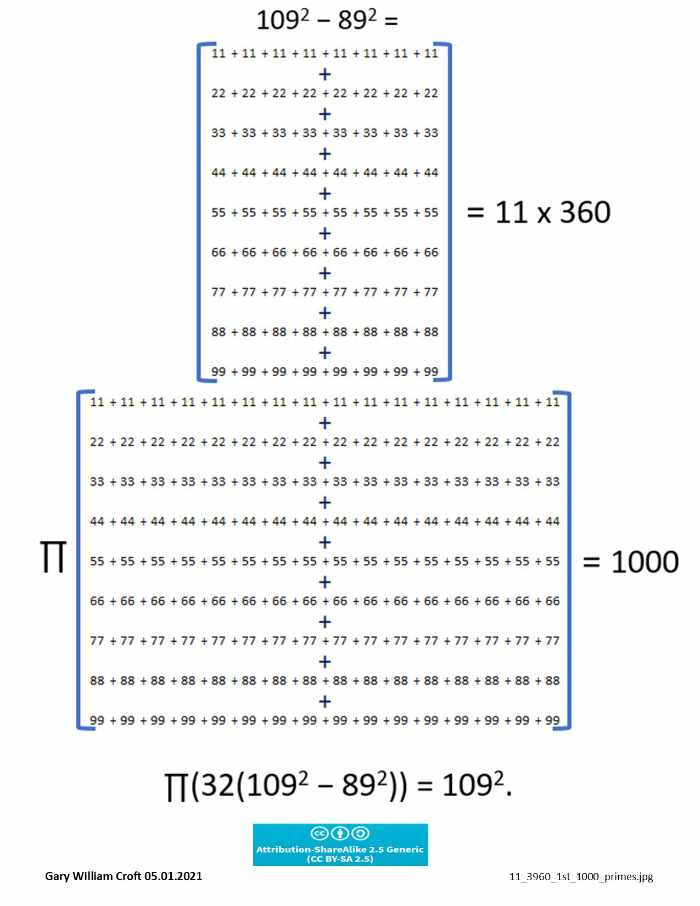By taking the correlation of these 11 partitions with the logical sequence of numbers there would be a linkage to the powers of 10.

Note

The first 1000 prime numbers are silently screaming: “Pay attention to us, for we hold the secret to the distribution of all primes!” We heard the call, and with ‘strange coincidences’ leading the way have discovered compelling evidence that the 1000th prime number, 7919, is the perfectly positioned cornerstone of a mathematical object with highly organized substructures and stunning reflectional symmetries. (PrimeDemystified)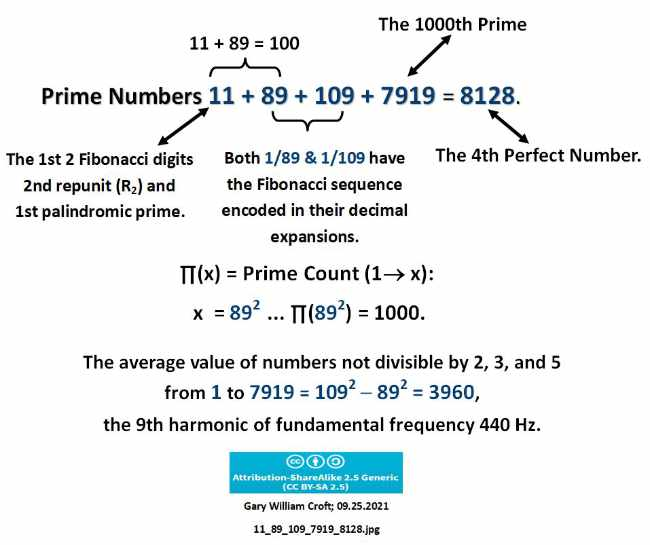Both 1/89 and 1/109 have the Fibonacci sequence in their decimal expansions illustrates a period-24 palindromic that bring the powers of pi.

## The powers of pi

This study cascade culminating in the Fibonacci digital root sequence (also period-24). So by simple words this 11 dimensions brings us back to the hexagonal formation.

Note

The spin states for the powers of pi. The Prime Hexagon is an integer environment, so pi powers are truncated. I believe these data suggest prime numbers are linked in some way to pi. (HexSpin)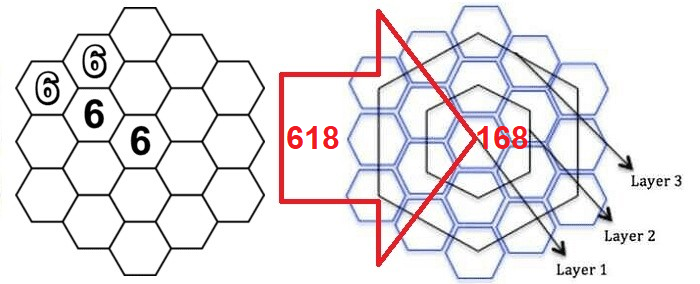Both scheme are carrying a correlation between two (2) number of 89 and 109 which provide the bilateral of 12 to the 24 cells of prime hexagon.

Note

In the matrix pictured below, we list the first 24 elements of our domain, take their squares, calculate the modulo 90 congruence and digital roots of each square, and display the digital root factorization dyad for each square (and map their collective bilateral 9 sum symmetry). (PrimesDemystified)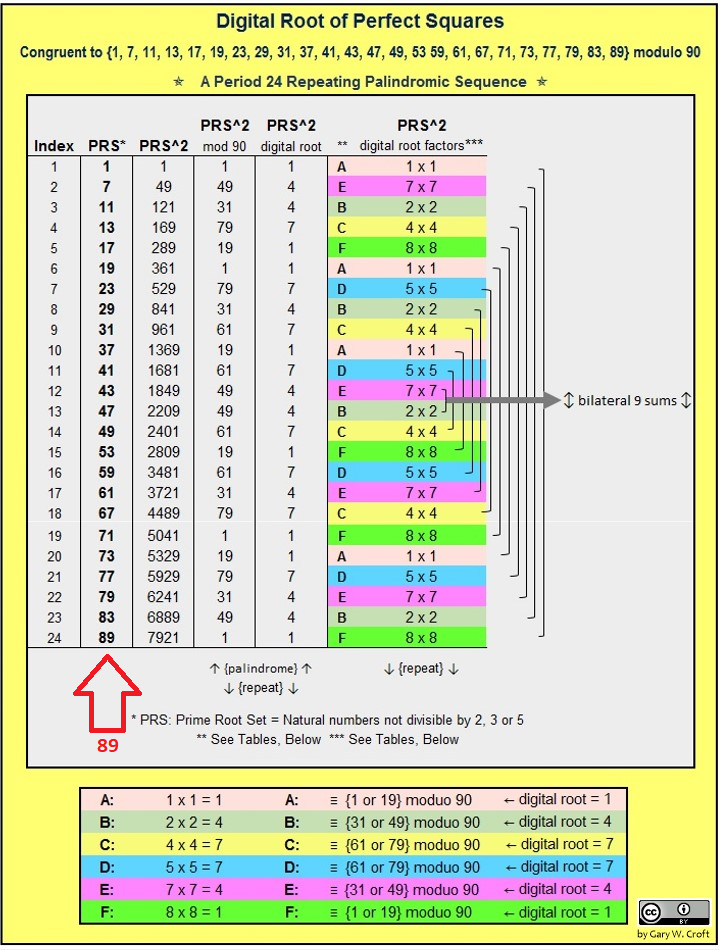Further observation we came to the conclusion that the behaviour of both scheme goes to seven (7) major partitions which is happen between the sequence of 30 to 36 while the twelve (12) is happen between the sequence of 36 to 102.

Tip

This behaviour finaly brings us to a suggestion that the dimension in string theory are linked with the prime distribution level as indicated by the self repetition on MEC30.

109 = 29th prime = (10th prime)th primeSo the 36 should behave as a central. Therefore the total files that inherited from this scheme will be `1 + 7 + 29 = 37` including one (1) main page.

This scheme will be organized via the Root Functions of each repositories.

## Δ(19 vs 18) Scenario

By our project, these 37 files are located within the wiki of main repository and organized by the 18's structure located per the 18 files of project gist.

Note

Every repository on GitHub.com comes equipped with a section for hosting documentation, called a wiki. You can use your repository’s wiki to share long-form content about your project, such as how to use it, how you designed it, or its core principles. (GitHub)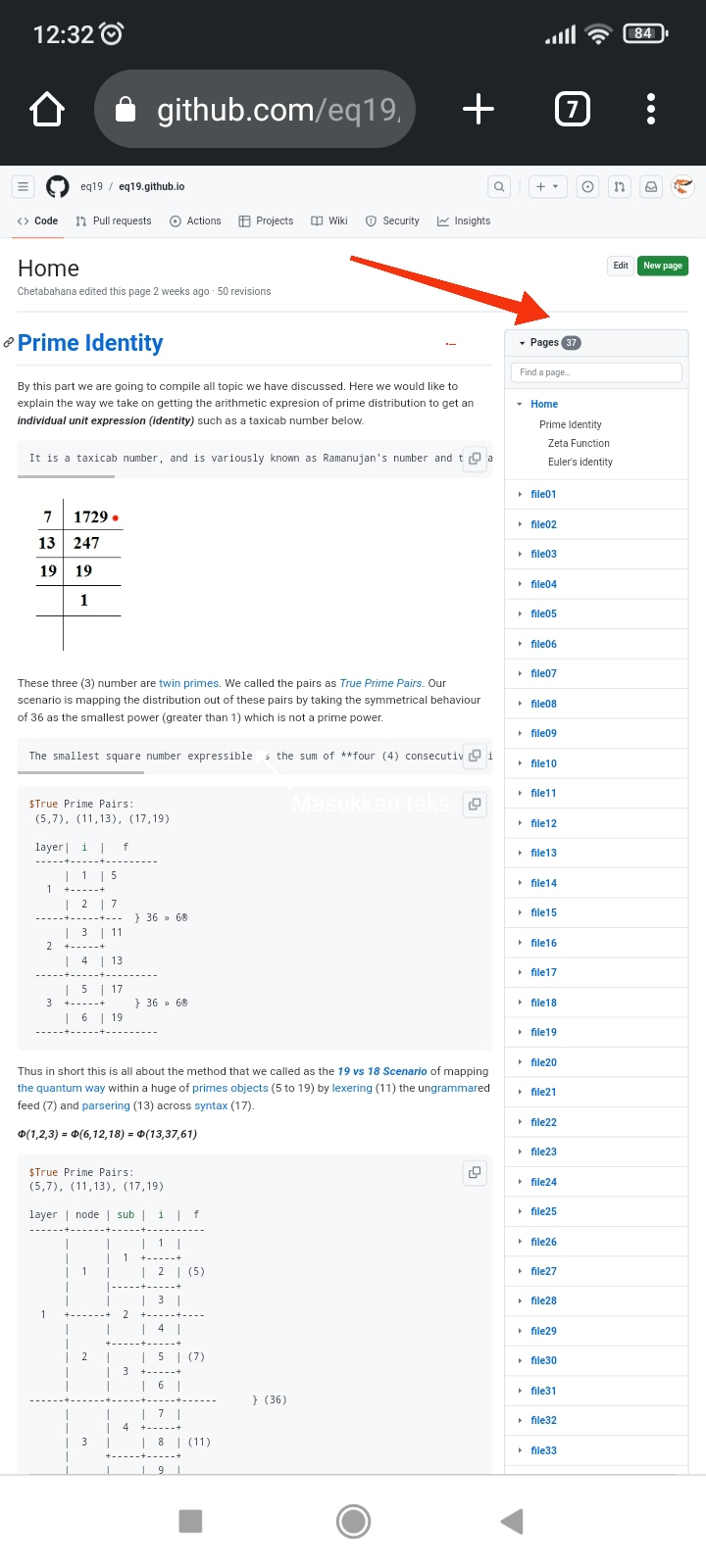Since we are discussing about prime distribution then this 18's structure will also cover the further scheme that is inherited from the above 37 files. We call this arrangement as Δ(19 vs 18) Scenario where the zeta function stands as the basic algorithm.

Note

This web enabled demonstration shows a polar plot of the first 20 non-trivial Riemann zeta function zeros (including Gram points) along the critical line Zeta(1/2+it) for real values of t running from 0 to 50. The consecutively labeled zeros have 50 red plot points between each, with zeros identified by concentric magenta rings scaled to show the relative distance between their values of t. Gram’s law states that the curve usually crosses the real axis once between zeros. (TheoryOfEverything)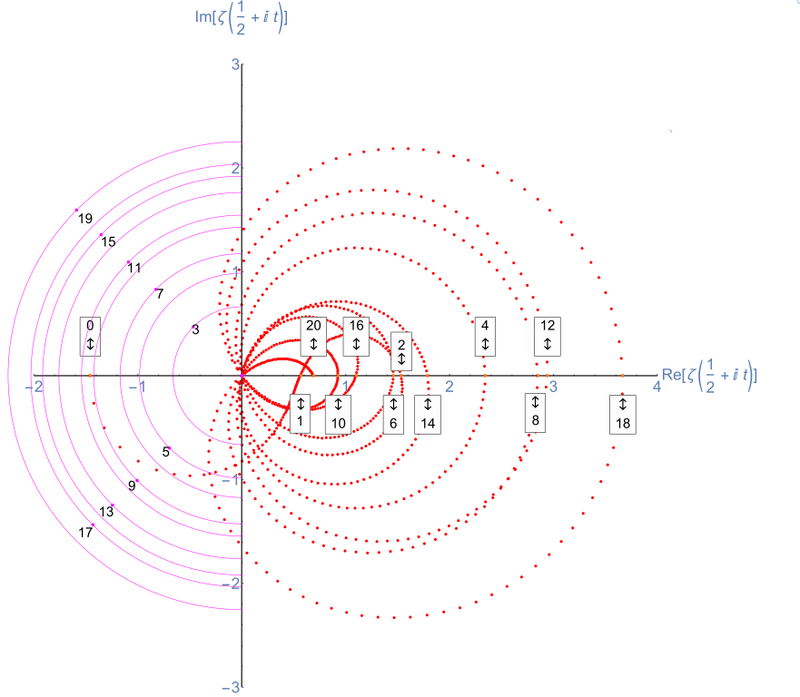When the seven (7) and eleven (11) are combined as eighteen (18) then ∆1 by the prime hexagon is recycled as nineteen (19) and generate `37 + 77 = 114` partitions.

Tip

By this tabulation you may see that all the numbers between 37 and 102 are located within 11 columns where the 31 behave as a new axis. So this 11 is reflecting the 19 to 29. Since the 11 is bonding with 19 so it would go to another cycles starting with the 26th dimension which will bring them by four (4) compactification (26 to 29) to the 30. This 30th order repeats itself to infinity. Even in the first 30s system.

``````  Δ1 + Δ7 + Δ29  →  | Δ37 + Δ77 = Δ114 = Δ113 + Δ1 →

|         1st (Form)          |         2nd (Route)         |         3rd (Channel)       |
-----+----+----+----+----+----+----+----+----+----+----+----+----+----+----+----+----+----+----+
150 | 151| 152| 153| 154| 155| 156| 157| 158| 159| 160| 161| 162| 163| 164| 165| 166| 167| 168|
=====+====+====+====+====+====+====+====+====+====+====+====+====+====+====+====+====+====+====+
Δ1 | 19 |  - | 31 | 37 |  - |  - |  - |  - |  - |  - |  - |  - |  - |  - | 103|  - |  - |  - |
+----+----+----+----+----+----+----+----+----+----+----+----+----+----+----+----+----+----+
Δ2 | 20 | 26 |  - | 38 |  - |  - |  - |  - |  - | 74 |  - |  - |  - | 98 | 104|  - |  - |  - |
-----+----+----+----+----+----+----+----+----+----+----+----+----+----+----+----+----+----+----+
Δ3 | 21 | 27 |  - | 39 |  - |  - |  - |  - |  - | 75 |  - |  - |  - | 99 | 105|  - |  - |  - |
+----+----+----+----+----+----+----+----+----+----+----+----+----+----+----+----+----+----+
Δ4 | 22 | 28 |  - | 40 |  - |  - |  - |  - |  - | 76 |  - |  - |  - | 100|  - |  - |  - |  - |
-----+----+----+----+----+----+----+----+----+----+----+----+----+----+----+----+----+----+----+
Δ5 | 23 | 29 |  - | 41 |  - |  - |  - |  - |  - | 77 |  - |  - |  - | 101|  - |  - |  - |  - |
+----+----+----+----+----+----+----+----+----+----+----+----+----+----+----+----+----+----+
Δ6 | 24 |  - |  - | 42 |  - | 54 |  - |  - | 72 | 78 |  - | 90 | 96 |  - |  - |  - |  - | 114|
=====+====+====+====+====+====+====+====+====+====+====+====+====+====+====+====+====+====+====+
Δ7 | 25 |  - |  - | 43 |  - | 55 |  - |  - | 73 | 79 |  - | 91 | 97 |  - |  - |  - |  - |  - |
+----+----+----+----+----+----+----+----+----+----+----+----+----+----+----+----+----+----+
Δ8 |  - |  - |  - | 44 |  - | 56 |  - |  - |  - | 80 |  - | 92 |  - |  - |  - |  - |  - |  - |
-----+----+----+----+----+----+----+----+----+----+----+----+----+----+----+----+----+----+----+
Δ9 |  - |  - |  - | 45 |  - | 57 |  - |  - |  - | 81 |  - | 93 |  - |  - |  - |  - |  - |  - |
+----+----+----+----+----+----+----+----+----+----+----+----+----+----+----+----+----+----+
Δ10 |  - |  - |  - | 46 | 52 | 58 |  - | 70 |  - | 82 | 88 | 94 |  - |  - |  - |  - | 112|  - |
-----+----+----+----+----+----+----+----+----+----+----+----+----+----+----+----+----+----+----+
Δ11 |  - |  - |  - | 47 | 53 | 59 |  - | 71 |  - | 83 | 89 | 95 |  - |  - |  - |  - | 113|  - |
+----+----+----+----+----+----+----+----+----+----+----+----+----+----+----+----+----+----+
Δ12 |  - |  - |  - | 48 |  - | 60 | 66 |  - |  - | 84 |  - |  - |  - |  - |  - | 108|  - |  - |
=====+====+====+====+====+====+====+====+====+====+====+====+====+====+====+====+====+====+====+
Δ13 |  - |  - |  - | 49 |  - | 61 | 67 |  - |  - | 85 |  - |  - |  - |  - |  - | 109|  - |  - |
+----+----+----+----+----+----+----+----+----+----+----+----+----+----+----+----+----+----+
Δ14 |  - |  - | 32 | 50 |  - | 62 | 68 |  - |  - | 86 |  - |  - |  - |  - |  - | 110|  - |  - |
-----+----+----+----+----+----+----+----+----+----+----+----+----+----+----+----+----+----+----+
Δ15 |  - |  - | 33 | 51 |  - | 63 | 69 |  - |  - | 87 |  - |  - |  - |  - |  - | 111|  - |  - |
+----+----+----+----+----+----+----+----+----+----+----+----+----+----+----+----+----+----+
Δ16 |  - |  - | 34 |  - |  - | 64 |  - |  - |  - |  - |  - |  - |  - | -  | 106|  - |  - |  - |
-----+----+----+----+----+----+----+----+----+----+----+----+----+----+----+----+----+----+----+
Δ17 |  - |  - | 35 |  - |  - | 65 |  - |  - |  - |  - |  - |  - |  - | -  | 107|  - |  - |  - |
+----+----+----+----+----+----+----+----+----+----+----+----+----+----+----+----+----+----+
Δ18 |  - | 30 | 36 |  - |  - |  - |  - |  - |  - |  - |  - |  - | -  | 102|  - |  - |  - |  - |
=====+====+====+====+====+====+====+====+====+====+====+====+====+====+====+====+====+====+====+
1  |  2 |  3 |  4 |  5 |  6 |  7 |  8 |  9 | 10 | 11 | 12 | 13 | 14 | 15 |  16|  17| 18 | 19 |
-----+----+----+----+----+----+----+----+----+----+----+----+----+----+----+----+----+----+----+
|       Δ    Δ    Δ           |                     Φ12     |       Δ                   Δ |
-113 +150 = +37                                             +102 = +139    -113 -114
``````

By the above tabulation, here you can see that the layout of our home page refers to the four (4) partitions of ∆1 i.e. id: 1-18, id: 19-30, id: 31-36, and id: 37-102.

Warning

Our gists would forms the 18s structure of 11s and 7s so whenever the 11s is streched to 4 dimensions it will be compacted by the 7s as extended branes and finally become another level of 11 dimensions which lead to the concept of multiple universes.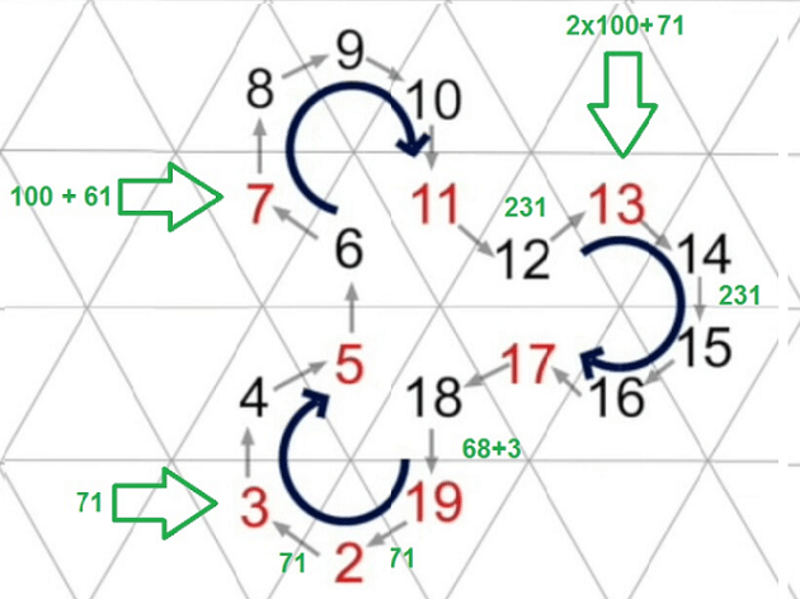So the four (4) zones is actually a streaching result by means of eigenvector. Thus it is the main reason why we see our live dimensions is four (4) instead of eleven (11).

Let's discuss how it goes by the seven (7) extra dimensions.

## Euler partition

By The Δ(19 vs 18) Scenario those three are exactly landed in the 0's cell out of Δ18. See that the sum of 30 and 36 is 66 while the difference between 36 and 102 is also 66.

Note

You likely noticed I began with 2 rather than 1 or 0 when I first constructed the hexagon. Why? Because they do not fit inside — they stick off the hexagon like a tail. Perhaps that’s where they belong. However, if one makes a significant and interesting assumption, then 1 and 0 fall in their logical locations – in the 1 and 0 cells, respectively. _(HexSpin)

0 + 30 + 36 + 102 = 168 = π(1000)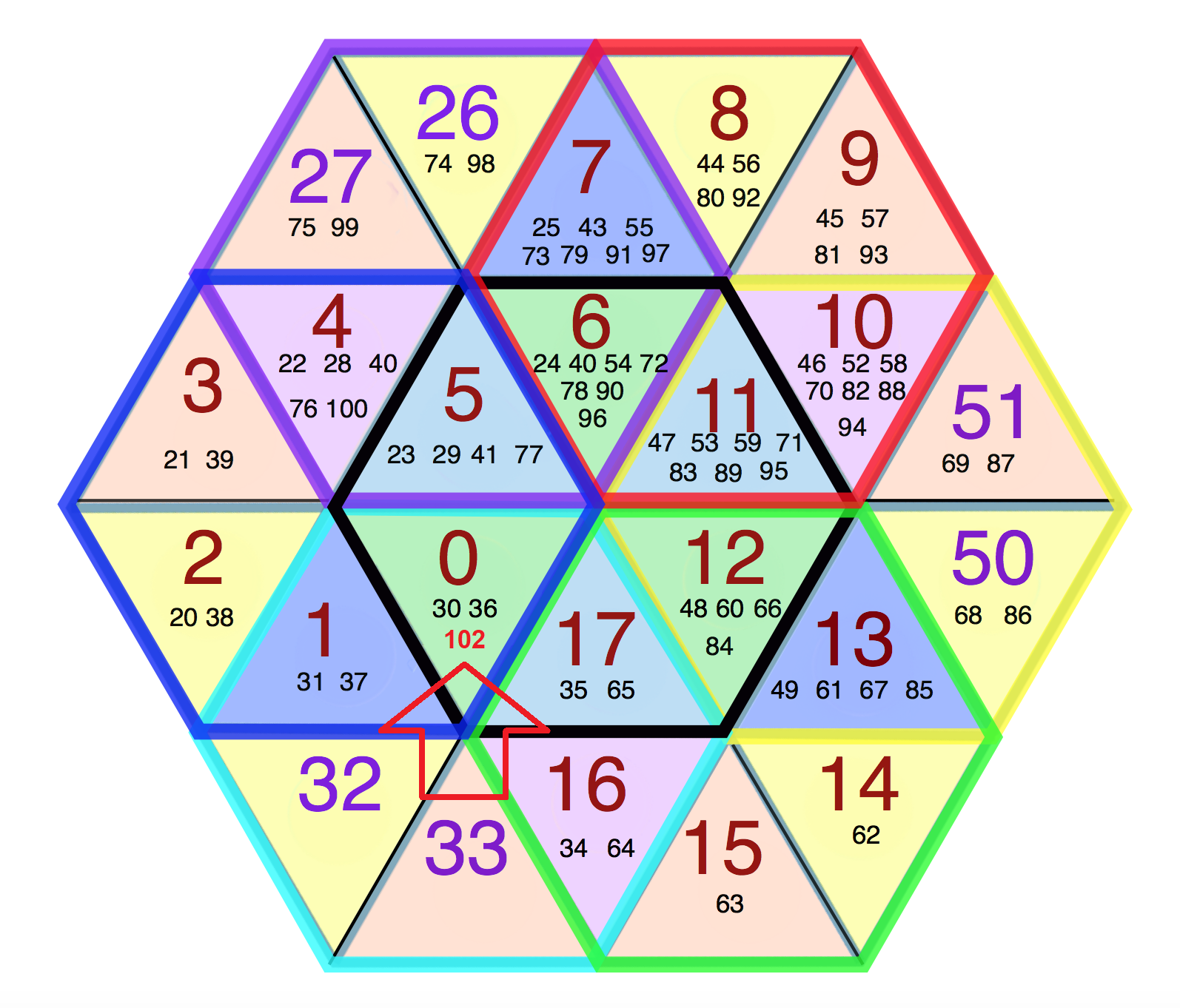The function that appears in the denominator is Partition Function. The equality between the product on the first line and the formulas in the third and fourth lines is Euler's pentagonal number theorem where `p(33) = 10143` landed exactly by `n - 7`.

Note

Using Euler’s method to find p(40): A ruler with plus and minus signs (grey box) is slid downwards, the relevant terms added or subtracted. The positions of the signs are given by differences of alternating natural (blue) and odd (orange) numbers. In the SVG file, hover over the image to move the ruler (Wikipedia).Here we can see that the transformation from 7 to 12 is actually started from the prime 13. So the power of 7 is transfered to 77 by the partition level of 29 and 89.

Note

We call this double strand a convolution, which results in an opposite arrangement. It represents the natural vibration, thus also the redundant vibrations in the energy transfer. In the 6 For example, in the graph, the even number 60 is folded. At folding of the even number 60 6 result in 8 prime pairs. (Google Patent DE102011101032A9)

89^2 - 1 = 7920 = 22 x 360 = 66 x 120 = (168 - 102) x 120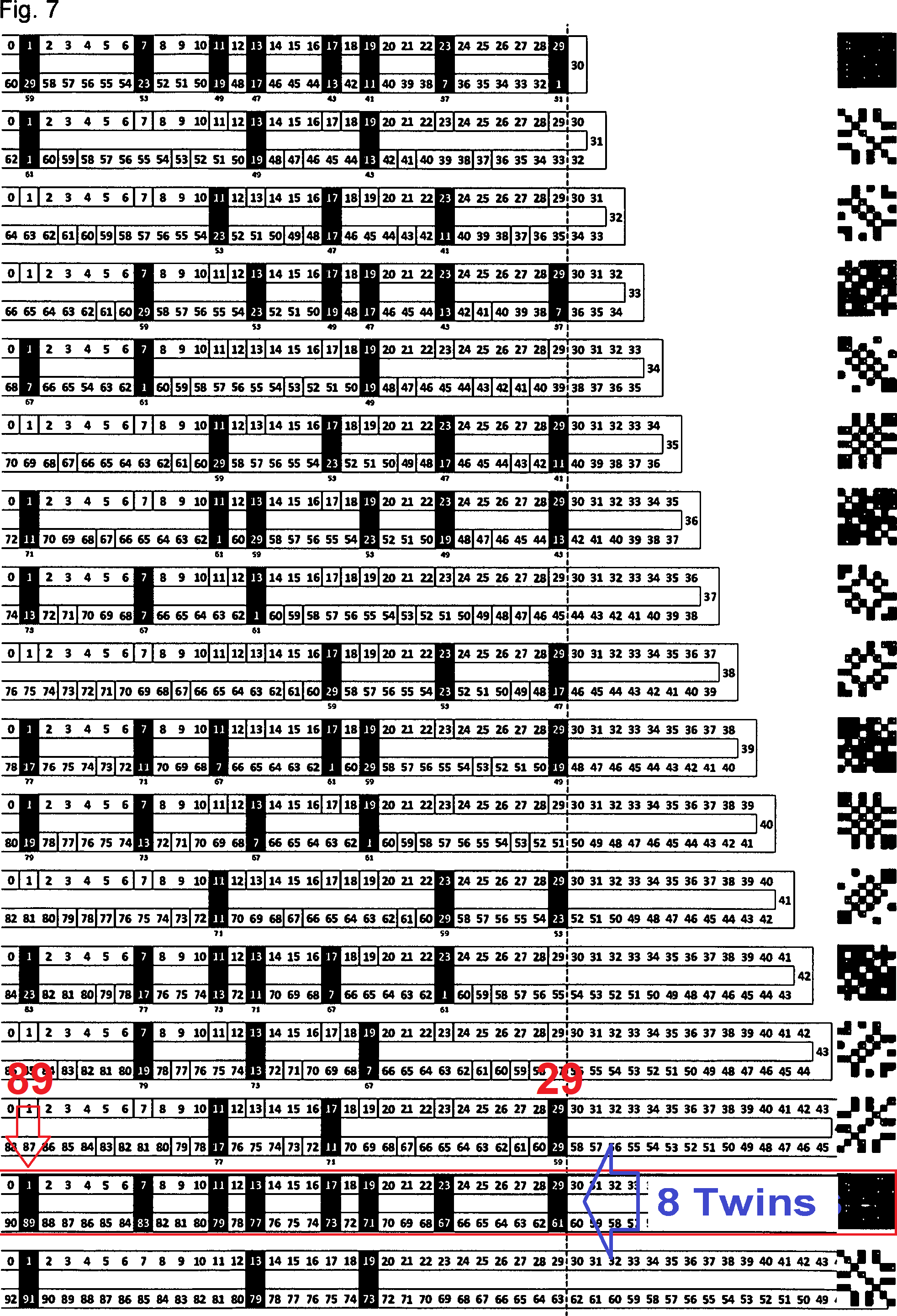As explicitly indicated by `n - 7` within identition zones this `p(33)` behave reversal to the exponentiation zones so it would stand as `π(π(π(1000th prime)))+1`. By the prime pair 11 and 13 the nodes is converted from 7 to 77 which is 7 times 11.

Note

Note that when 77 contains ‘Lucky 7 and 11’ as prime factors it is also the product of the middle two numbers of this sequence (11*7 = 77). (Prime Curios!)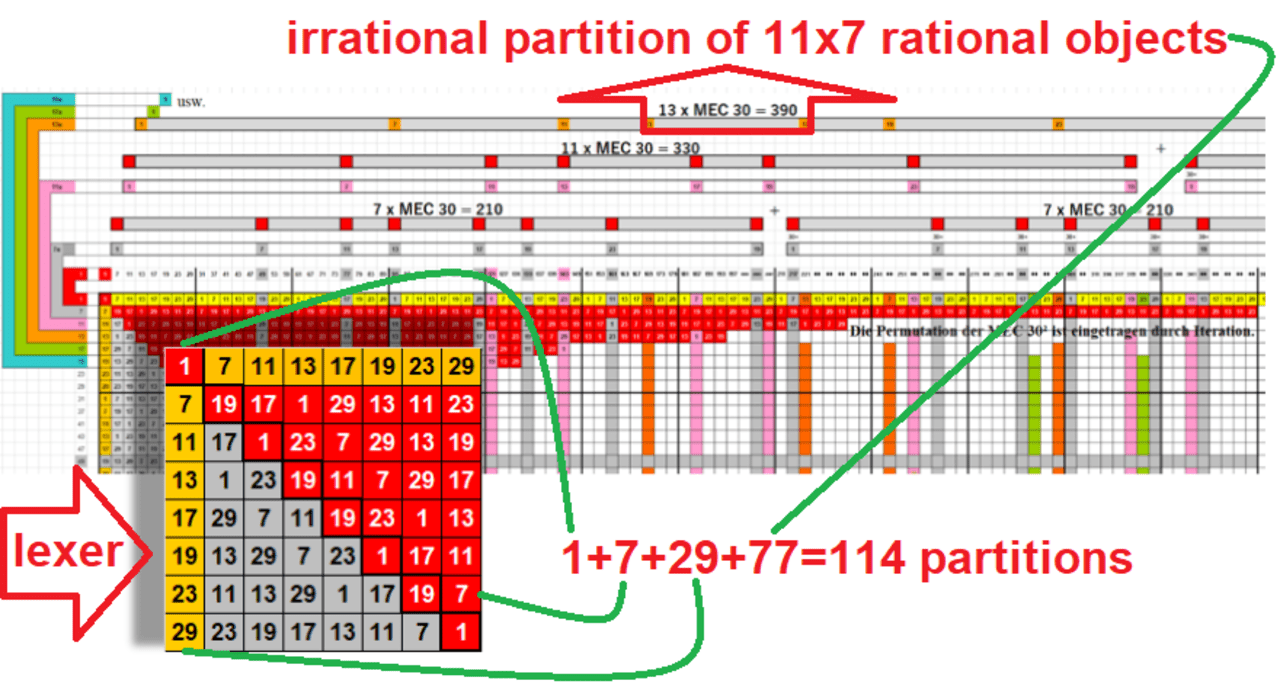By the next term there are fractions within these 1000 primes which end up with 168 identities of the same structure as the seven (7) pàrtitions of addition zones.

The only difference is the base unit of the lexer `(Id: 33)`.
It is now carrying the above `p(33) = 10143`.